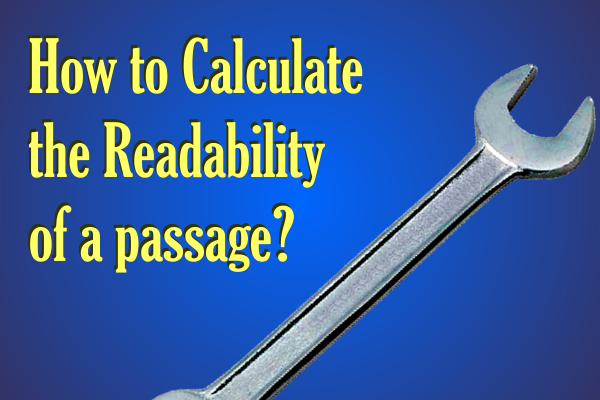## Apr 6, 2015

### How to calculate the readability of a passageThe engine at read-able.com performs its function flawlessly when you add some text to be analyzed.

The following text is from an article on "4 crucial facts Google Analytics can tell about your readers". And the following are the results after assessing the piece of text.

As can be seen from the table itself, several parameters are calculated on the text. An explanation with the formula for each parameter can be found below.

## Simple Textual Properties of the passage

Text Statistics Value
No. of sentences 7
No. of words 163
No. of complex words 16
Percent of complex words 9.82%
Average words per sentence 23.29
Average syllables per word 1.45

No. of sentences
Simply counting the number of full stops along with an algorithm

No. of words
Simply counting the number of spaces along with an algorithm

No. of complex words
I have no Idea, what can be construed as a complex word and what cannot. In my opinion, there must be a threshold number of characters in a word, exceeding which; the word can be construed as a complex word.

Percent of complex words
It can be calculated with the ratio of number of complex words to the number of total words.
The following is a valid formula for the same,
Percentage of Complex Words = ( ( number of complex words / Total Number of Words ) x 100 )

Average words per sentence
It can be calculated simply by dividing the number of words with the number of sentences
Average Words Per sentence = ( Number of Words / Number of sentences )

Average syllables per word
Syllable is a single unit of a word, separated in accordance with its sound. If you type 'Gutter" you have two syllables "Gut" and "ter". So the word "Gutter" will have two syllables.
A valid formula for the same can be

## Scores found through empirical Formulas

Gunning Fog Score 13
SMOG Index 8.8
Coleman Liau Index 11.1

Flesch–Kincaid Scores
Flesch–Kincaid readability test indicates the difficulty in understanding a text. They use word length and sentence length as parameters for their calculations.
The scale ranges from 0 to 100. Higher the score, easier is the text to read. If you receive a really low score, it means that the text is really complicated and hard to understand.
Flesch–Kincaid Score = 206.835 - 1.015 x (Words/sentences) - 84.6 x (Syllables/words)

Gunning Fog Score
Gunning Fog Index is simply the number of years of formal study; a typical US student would have undergone to understand the text written. So if you get a fog index of 11, it would mean that a typical US student of about 17 years would comprehend the subject completely.
The following is the formula for the same.
Fog Index = 0.4 x ( (Words/sentences) + 100 x (complex Words/Words) )

SMOG is a short form of Simple Measure of Gobbledygook. Just as Fog index measured the years of schooling required to interpret a piece of text for US based students, SMOG index can be applied anywhere.
The following is a formula for the same.
SMOG Index = 1.0430 x square root (30 x complex Words/sentences) + 3.1291

Coleman–Liau index
This index is also used to determine the difficulty of text. It uses only the number of letters per 100 words and the average number of sentences per 100 words.
The following is a formula for the same.
Coleman–Liau index = 0.0588(letters per 100 words) - 0.296(sentences per 100 words) - 15.8